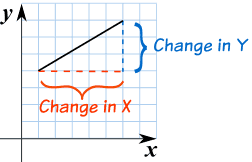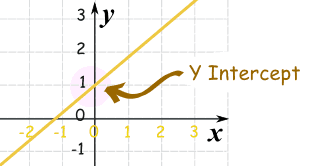### y = mx + b

m = Slope (how steep the line is)

b = the Y Intercept (where the line crosses the Y axis)\### How do you find “m” and “b“?

• b is easy: just see where the line crosses the Y axis.
• m (the Slope) needs some calculation. Remember we like to write slope like a fraction.

## Example 1)The fastest and easiest thing to find first when looking at a graph is the y-intercept (b).

Here we see that the line crosses the y-axis at positive 1.

So, b = 1 .

Now to find the slope (m) we will use rise over run:

• Rise is how far up
• Run is how far along

In this example the rise is 2 and the run is 1.

So, m = 2/1 .

Now that I know m = 2/1 and b = 1 I can plug them into the equation for slope intercept form y = mx + b.

y = (2/1) x + 1

^^^^^ This is the equation of the line.

# SLOPE

Standard

Slope is about how steep a line is.
When finding the slope from a line on a graph we use the method of rise over run .
• Rise is how far up
• Run is how far alongYou can also think of it as the change in y over the change in x.EXAMPLE #1:The slope here is 4/6 which can simplify to 2/3.
EXAMPLE #2:In this example the slope is 3/5.

# Practice Problems

TEST YOUR SELF ON LINES HERE!!!!

# Y – INTERCEPT

Standard

## Y Intercept

Where a straight line crosses the Y axis of a graph.

### Example:In the above diagram the line crosses the Y axis at 1.

So the Y intercept is equal to 1.

## Practice Problems

TEST YOUR SELF ON LINES HERE!!!!

# Standard Form of a Linear Equation:

The “Standard Form” for writing down a Linear Equation is

Ax + By = C

A shouldn’t be negative, A and B shouldn’t both be zero, and A, B and C should be integers.

### y = 3x + 2

Bring 3x to the left:

-3x + y = 2

Multiply all by -1:

3x – y = -2

Note: A=3, B=-1, C=-2

This form:

Ax + By + C = 0

is sometimes called “Standard Form”, but is more properly called the “General Form”.

ALGEBRA REVIEW KAHOOT:

https://play.kahoot.it/#/?quizId=8af8cbf5-78da-4760-8b40-ccc70cd54a6e

# Slope Kahoot:

https://play.kahoot.it/#/k/31ab2ba7-803c-45a4-b504-ccc96ce3ea17

# Y-Intercept Kahoot:

https://play.kahoot.it/#/k/e4aa37b7-7aac-4f4c-9d8c-4fe790b1c78d